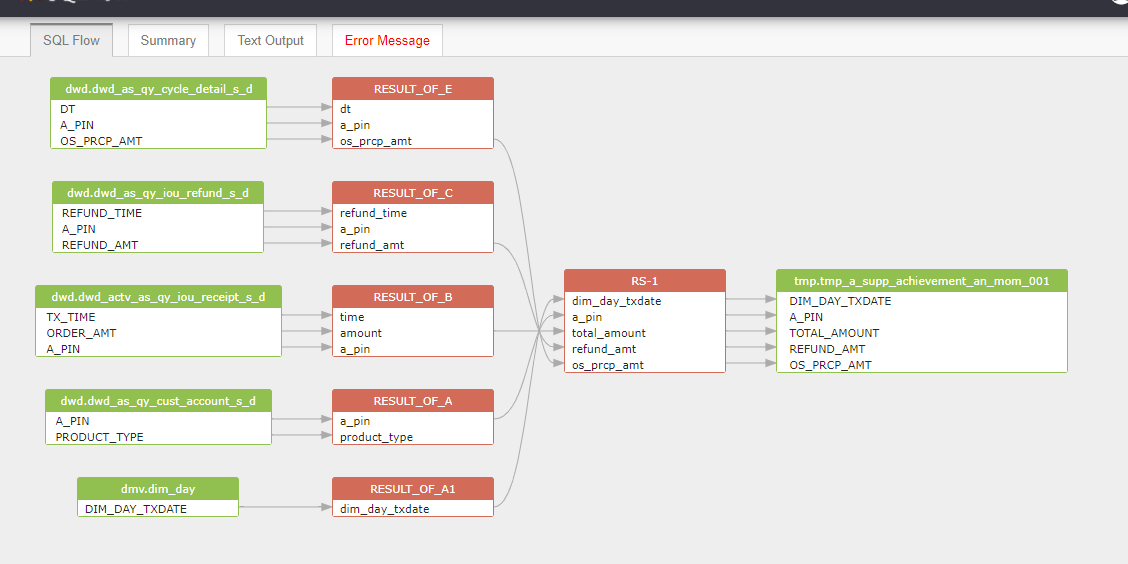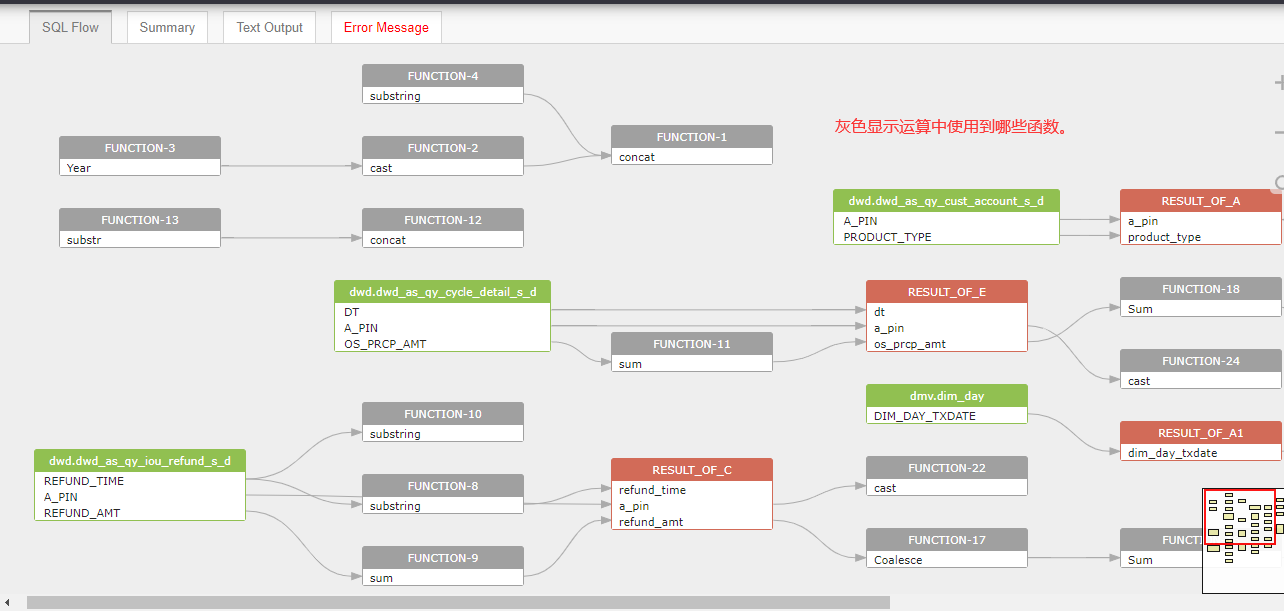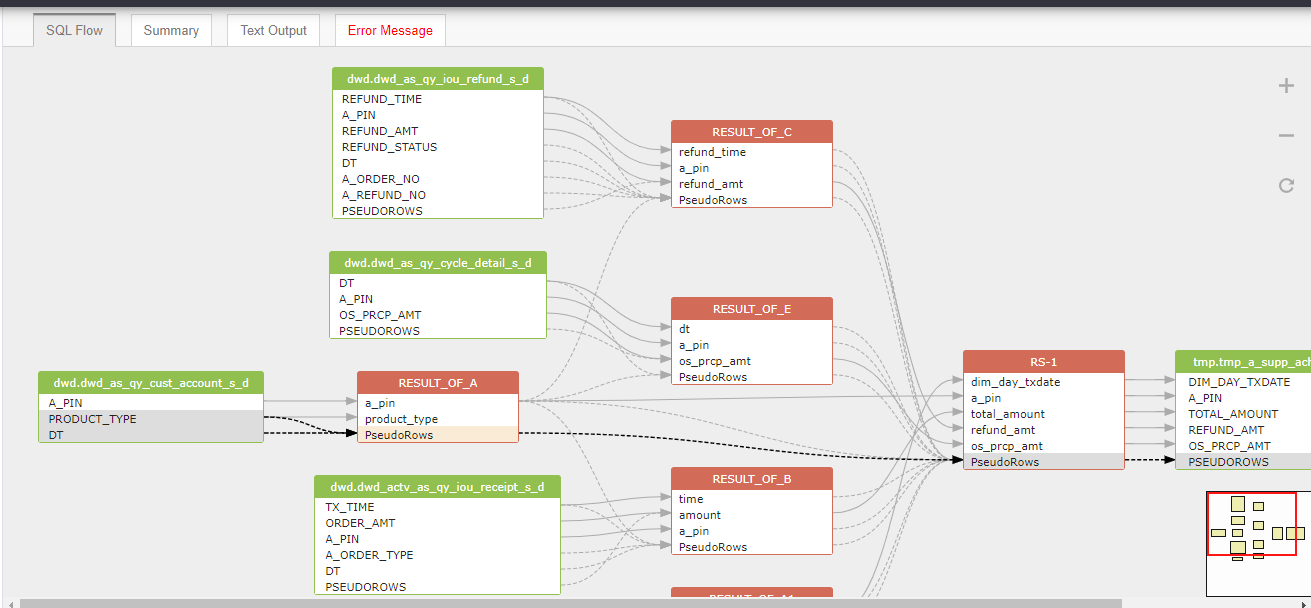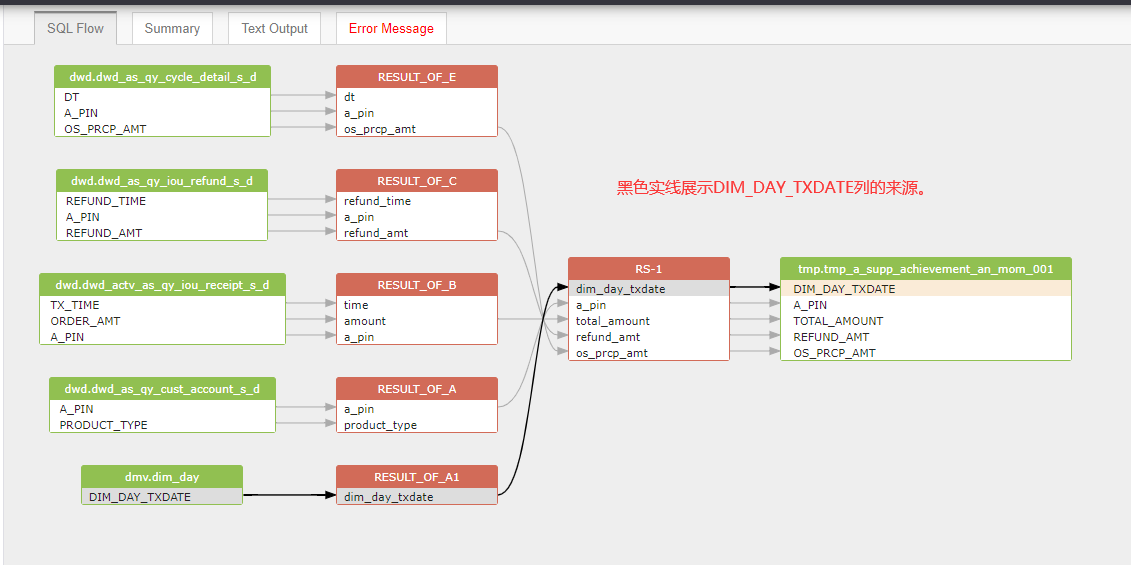# 血缘关系分析工具SQLFLOW--实践指南

2021-05-29 22:57 162 查看

SQLFlow 是用于追溯数据血缘关系的工具，它自诞生以来以帮助成千上万的工程师即用户解决了困扰许久的数据血缘梳理工作。

SQLFlow 通过分析各种数据库对象的定义(DDL)、DML 语句、ETL/ELT中使用的存储过程(Proceudre,Function)、 触发器(Trigger)和其他 SQL 脚本，给出完整的数据血缘关系。

今日根据mysql的一个实例SQL进行进一步介绍该工具的具体操作：

```MYSQL SQL:CREATE TABLE tmp.tmp_a_supp_achievement_an_mom_001 AS
SELECT a1.dim_day_txdate,
a.a_pin,
Sum(Coalesce(b.amount, 0)) AS total_amount
, Sum(Coalesce(c.refund_amt, 0)) AS refund_amt
, Sum(os_prcp_amt) os_prcp_amt
FROM (SELECT dim_day_txdate
FROM dmv.dim_day
WHERE dim_day_txdate>=concat(cast(Year('2018-05-15')-1 AS string),'-', substring('2018-05-15', 6, 2), '-01')
AND dim_day_txdate<='2018-05-15' )a1
JOIN (SELECT DISTINCT a_pin, product_type
FROM dwd.dwd_as_qy_cust_account_s_d
WHERE dt ='2018-05-15' AND product_type='20288' )a
LEFT OUTER JOIN (SELECT substring(tx_time, 1, 10) AS time, sum(order_amt) AS amount, a_pin
FROM dwd.dwd_actv_as_qy_iou_receipt_s_d
WHERE a_order_type='20096' AND a_pin NOT IN ('vep_test', 'VOPVSP测试')
AND dt='2018-05-15'
GROUP BY substring(tx_time, 1, 10), a_pin )b
ON cast(a.a_pin AS string)=cast(b.a_pin AS string) AND a1.dim_day_txdate=b.time
LEFT OUTER JOIN ( SELECT substring(refund_time, 1, 10) AS refund_time, a_pin, sum(refund_amt)AS refund_amt
FROM dwd.dwd_as_qy_iou_refund_s_d
WHERE refund_status='20090' AND dt='2018-05-15' AND a_order_no <> '12467657248'
AND a_refund_no <> '1610230919767139947'
GROUP BY substring(refund_time, 1, 10), a_pin )c
ON cast(a.a_pin AS string)=cast(c.a_pin AS string) AND a1.dim_day_txdate=c.refund_time
LEFT OUTER JOIN (SELECT dt, a_pin, sum(os_prcp_amt) AS os_prcp_amt
FROM dwd.dwd_as_qy_cycle_detail_s_d
WHERE dt>=concat(substr('2018-05-15', 1, 7), '-01') AND dt<='2018-05-15'
GROUP BY dt, a_pin)e
ON cast(a.jd_pin AS string)=cast(e.a_pin AS string) AND a1.dim_day_txdate=e.dt
GROUP BY a1.dim_day_txdate, a.a_pin;```

• SQLFlow是怎么样工作的

SQLFlow通过自动分析SQL逻辑关系，能够快速、准确的识别出SQL语句中各对象的关联关系及每个对象参与运算的列名称，如果存在多级运算，它的展示还包含各级的中间运算集合，让你能够清晰的阅读，非常适合阅读。• 显示SQL语句使用了哪些函数• 显示对象间的关联关系

JOIN (SELECT DISTINCT a_pin, product_type
FROM dwd.dwd_as_qy_cust_account_s_d
WHERE dt ='2018-05-15' AND product_type='20288' )a• 血缘关系追踪

从上面的sql中，进行我们的解析可以得到dim_day_txdate字段来源于dmv.dim_day，a_pin来源于dwd.dwd_as_qy_cust_account_s_d，total_amount是 来源于DWD.dwd_actv_as_qy_iou_receipt_s_d中的(order_amt)经过sum之后得到amount 再通过sum和coleace操作得到的，这系列的血缘关系变化我们将用链表方式进行存储，得到最终的血缘关系。关于当前比较流行的SQLFlow工具是如何分析 mysql 语句， 56c 从而得到怎样的表、字段间的数据血缘关系 data lineage的内容就先分享到这里，后续精彩继续~~~